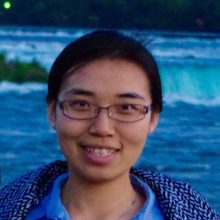# Yuqian Zhang

•Yuqian Zhang

Ph.D. Candidate, Columbia University
Email

Abstract
Geometry and Algorithm of Sparse Blind Deconvolution

Blind deconvolution is the problem of recovering a convolutional kernel and an activation signal from their convolution. This problem is ill-posed without further constraints or priors. We study the short-and-sparse blind deconvolution problem where the convolutional kernel is short, and the activation signal has sparsely and randomly populated nonzero entries. We normalize the convolution kernel to have unit Frobenius norm and cast the sparse blind deconvolution problem as a nonconvex optimization problem over the sphere. With such formulation, every spurious local minimum turns out to be close to some signed shift truncation of the ground truth, under certain hypotheses. Based on this benign geometric property, we propose several effective algorithms that recover the ground truth. Moreover, both the geometric characterization of local solutions and the proposed algorithms carry over to convolutional dictionary learning problem, where the observation is a superposition of multiple pairs of convolutional signals.

Bio
Yuqian Zhang is a Ph.D. candidate in the Electrical Engineering Department at Columbia University and is advised by Professor John Wright. She received her B.S. in Electrical Engineering from Xi’an Jiaotong University. Her research spans across optimization, computer vision, signal processing, and machine learning. Specifically, her primary research interest is to develop efficient, reliable and robust algorithms for applications in computer vision, scientific data analysis, etc.